## Numerical Ability

#### Numerical Ability

1. X bullocks and Y tractors take 8 days to plough a field. If we halve the number of bullocks and double the number of tractors, it takes 5 days to plough the same field. How many days will it take X bullocks alone to plough the field?
1. 30
2. 35
3. 40
4. 45

1. Let the total work be 40 units i.e. LCM of 8 and 5 days.
x + y = 40/8.... (i)
and (x/2) + 2y = 40/5 ....(ii)
Solving (i) and (ii) we get x = 4/3.
Hence, x alone can do the work in 30 days

##### Correct Option: A

Let the total work be 40 units i.e. LCM of 8 and 5 days.
x + y = 40/8.... (i)
and (x/2) + 2y = 40/5 ....(ii)
Solving (i) and (ii) we get x = 4/3.
Hence, x alone can do the work in 30 days

1. A wire of length 340 mm is to be cut into two parts. One of the parts is to be made into a square and the other into a rectangle where sides are in the ratio of 1 :2. What is the length of the side of the square (in mm) such that the combined area of the square and the rectangle is a MINIMUM?
1. 30
2. 40
3. 120
4. 180

1.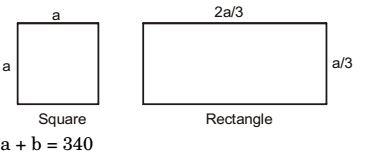Perimeter of rectangle = 2a + 2a= 2a 3 3

 Length of square = 340 - 2a 4

 Total area =340 - 2a² + 2ae = f'(a) 4 4

Condition for minimum area f'(a) = 0
 2340 - 2a- a+ 4 a = 0 4 2 9

 4 a = 1 (340 - 20) 9 4

a = 90
 Length of the square = 340 - 2a = 40 mm 4

##### Correct Option: BPerimeter of rectangle = 2a + 2a= 2a 3 3

 Length of square = 340 - 2a 4

 Total area =340 - 2a² + 2ae = f'(a) 4 4

Condition for minimum area f'(a) = 0
 2340 - 2a- a+ 4 a = 0 4 2 9

 4 a = 1 (340 - 20) 9 4

a = 90
 Length of the square = 340 - 2a = 40 mm 4

1. Given (9 inches)1/2 = (0.25 yards)1/2, which one of the following statements is TRUE?
1. 3 inches - 0.5 yards
2. 9 inches = 1.5 yards
3. 9 inches = 0.25 yards
4. 81 inches = 0.0625 yards

1. 9 inches = 0.25 yards will be the right answer. Option (a) and (d) are wrong because we need to do the square root and square of not just the numbers but the units as well i.e. inches and yards can't remain the same.

##### Correct Option: C

9 inches = 0.25 yards will be the right answer. Option (a) and (d) are wrong because we need to do the square root and square of not just the numbers but the units as well i.e. inches and yards can't remain the same.

1. Which of the following curves represents the function y = (|esinC( |x|)) for |x| < 2π ?
Here, x represents the abscissa and y repreents the ordinate.
1.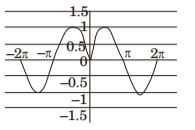2.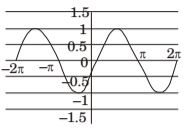3.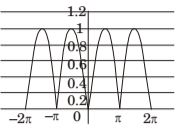4.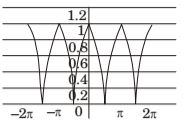1. f(x) = ln(| e[| sin(| x|)|]|)
1. |x| ⇒ f(x) is Even. Hence, option (b) is not possible
2. m =| sin(|x| )| > = 0 ⇒ em > = 1
3. ⇒f(x) = ln(|em|) = ln(em) >= 0 {from 2}
Hence, option (a) is not possible
4. and f(x)x=0 = 0
Hence, option (d) is not possible
Therefore, correct option is (c).

##### Correct Option: C

f(x) = ln(| e[| sin(| x|)|]|)
1. |x| ⇒ f(x) is Even. Hence, option (b) is not possible
2. m =| sin(|x| )| > = 0 ⇒ em > = 1
3. ⇒f(x) = ln(|em|) = ln(em) >= 0 {from 2}
Hence, option (a) is not possible
4. and f(x)x=0 = 0
Hence, option (d) is not possible
Therefore, correct option is (c).

1. Find the missing sequence in the letter series. B, FH, LNP, ________.
1. SUWY
2. TUVW
3. TVXZ
4. TVVXZ

1. The following letter series is in the order of even letters series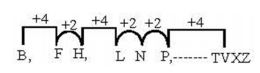##### Correct Option: C

The following letter series is in the order of even letters series